# Quiz 9: Measuring and Managing Real Exchange Risk

Inflation of two countries B and A are provided in order to determine the percentage strengthening and weakening of Country A currency peso in real terms: Following are the synopsis of given information:If the Brazil real is weakening by 21% then the Country A currency will appreciate by 26.58% calculate it as follows:Thus, the appreciation in currency A will be 26.58%. Here use the relative change in the nominal exchange rate that is measured between currency of country B that is real per country A currency peso. Below formula is used to determine the change in the real exchange. Determination of Change in the Real exchange rate can be made using the below formula:On substituting the variables in the above equation:In real terms country A peso (currency) has weakened by 17.41% which is lesser than the nominal rate of 21%. The main reason for this is that the inflation in country A is greater than Country B only by 5% (i.e. 15% - 10%). The above calculation it is clear that the competitiveness of the country A has actually not improved because the given weakness of the currency is higher than the real weakness and the inflation of Country A is greater than Country B. This clearly indicates that Country A is losing its competitiveness in the international trade and it will not be attractive for the international players to trade with this country. Country A has to work on the inflation which is higher in order to maintain the trade competitiveness.
A domestic production unit supply product in domestic country and in foreign market. Demand for both the market is being provided in order to determine the optimal quantity and prices to be charged in both the markets. Domestic market demand: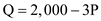Foreign market demand: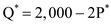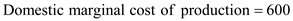Step 1: Domestic market: All variables are as per given information. First using the domestic equation we determine the value of P which is the price.Revenue from the domestic sales is determined by multiplying the price per unit and the quantity sold.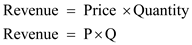In this case, we can substitute the value of P in the revenue equation:Differentiating the equation with respective to Q: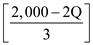Step 2: Foreign market: In case of monopolistic market will sell the output in foreign market, then the domestic revenue in terms of real value from the foreign country sales in real exchange rate which is determined by multiplying RS with the foreign market revenue that is determined as domestic market. First using the domestic equation we determine the value of P which is the price.Revenue from the domestic sales is determined by multiplying the price per unit and the quantity sold.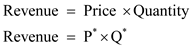In this case, we can substitute the value of P in the revenue equation:Let us multiply RS in both side of the above equation: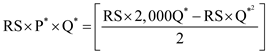Step 3: Calculation of price per unit: All variables are as per Step 1, Step 2 and given information. The total production cost is determined by multiplying the per unit cost of production with the total quantity that is both domestic and foreign country sales quantity. The same can be expressed as the below equation.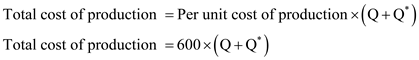The pricing strategy of a monopolistic player will be such that the marginal revenues earned by way of sales in each of the market will be equivalent to common marginal costs. Thus, the quantity to be sold in domestic market can be determined by equating the revenue and the marginal cost that is 600.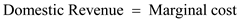On substituting the variables in the above expression:On substituting the value of Q in the price equation will result in the price per unit: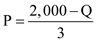On substituting the value of Q: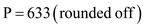Step 4: Calculation of total real profit: All variables are as per Step 1 , Step 2 , Step 3 and given information. The equation of marginal revenue from the foreign market is as follows: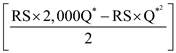Differentiating with respective to Q * :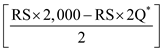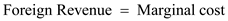On substituting the variables in the above expression: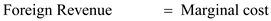As per the given information the real exchange rate is 1 which indicates that RS will be eliminated and Q will be determined.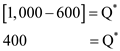On substituting the value of Q in the price equation will result in the price per unit: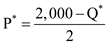On substituting the variables in the above equation: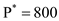Total real profit total revenue from both the domestic and foreign market minus the cost. Real profit = [P * Q] + [P * * Q * ] - 600[Q+Q * ] On substituting the variables in the above equation:Step 5: All variables are as per Step 1 , Step 2 , Step 3 , Step 4 and given information: As per the given information, there is a real appreciation in the exchange rate by 10%, then the new real exchange rate will be 1.10 (i.e. 1×(1+0.10)). This appreciation will be beneficial to the exporting monopolist. The foreign marginal revenue will change but the marginal cost will remain constant equating both will result in new quantity to be sold.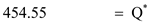On substituting the value of Q in the price equation will result in the price per unit:On substituting the variables in the above equation: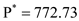Total real profit is; total revenue from both the domestic and foreign market minus the costs. Real profit = [P * Q] + [P * * Q * ] - 600[Q+Q * ] On substituting the variables in the above equation:The ratio of new price by old price in the foreign market is as follows: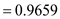The ratio of decrease in price is comparatively lesser than the change in the currency rate. Though, production can be increased.